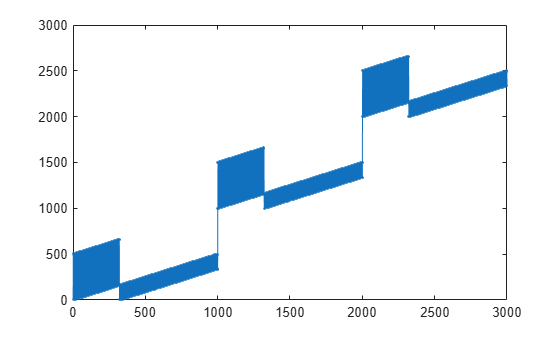# nrRateMatchLDPC

Low-density parity-check (LDPC) rate matching

## Syntax

``out = nrRateMatchLDPC(in,outlen,rv,mod,nLayers)``
``out = nrRateMatchLDPC(___,Nref)``

## Description

example

````out = nrRateMatchLDPC(in,outlen,rv,mod,nLayers)` returns the rate-matched output of length `outlen` for input data matrix `in`. The input `rv` is the redundancy version, `mod` is the modulation type, and `nLayers` is the number of transmission layers. The internal buffer used for the soft input has no size limits.`nrRateMatchLDPC` includes the stages of bit selection and interleaving defined for LDPC-encoded data and code block concatenation, as specified in TS 38.212 Sections 5.4.2 and 5.5 .```
````out = nrRateMatchLDPC(___,Nref)` returns the rate-matched output for a limited soft buffer size `Nref`, in addition to the input arguments in the previous syntax. `Nref` is defined in TS 38.212 Section 5.4.2.1 .```

## Examples

collapse all

Create input data corresponding to two LDPC-encoded code blocks of length 3960.

`encoded = ones(3960,2);`

Perform LDPC rate matching of the two code blocks to a vector of length 8000. Use single transmission layer with QPSK modulation and zero redundancy version.

```rv = 0; mod = 'QPSK'; nLayers = 1; outlen = 8000; ratematched = nrRateMatchLDPC(encoded,outlen,rv,mod,nLayers); size(ratematched)```
```ans = 1×2 8000 1 ```

Create LDPC-encoded input data consisting of integer ramps in separate code blocks. Perform LDPC rate matching of the code blocks to a vector of length 3000. Use single transmission layer with QPSK modulation and zero redundancy version. Plot the locations of the rate-matched output data.

```encoded = [0 1000 2000] + (1:66*10)'; rv = 0; mod = 'QPSK'; nLayers = 1; outlen = 3000; out = nrRateMatchLDPC(encoded,outlen,rv,mod,nLayers); plot(out,'.-')```## Input Arguments

collapse all

LDPC-encoded input data, specified as a matrix. Each column of `in` is a codeword. The number of columns in the input argument `in` is equal to the number of scheduled code blocks in a transport block. Each column is rate-matched separately, and the results are concatenated in `out`.

Data Types: `double` | `int8`

Length of the rate-matched and concatenated output vector, specified as a positive integer. `outlen` is the number of coded bits available for transmission in the transport block, as specified in TS 38.212 Section 5.4.2.1 .

The modulation scheme `mod` determines the modulation order ${Q}_{m}$(number of bits used per modulation symbol). If `outlen` is not a multiple of `nLayers` × ${Q}_{m}$, the function sets the length of the output vector to the next multiple of `nLayers` × ${Q}_{m}$.

Data Types: `double`

Redundancy version, specified as an integer from 0 to 3.

Data Types: `double`

Modulation scheme, specified as `'pi/2-BPSK'`, `'QPSK'`, `'16QAM'`, `'64QAM'`, or `'256QAM'`. This modulation scheme determines the modulation type of the codeword and the number of bits used per modulation symbol.

Modulation SchemeNumber of Bits Per Symbol

`'pi/2-BPSK'`

1
`'QPSK'`2
`'16QAM'`4
`'64QAM'`6
`'256QAM'`8

Data Types: `char` | `string`

Number of transmission layers associated with the transport block, specified as an integer from 1 to 4.

Data Types: `double`

Limited buffer rate matching, specified as a positive integer. `Nref` is defined in TS 38.212 Section 5.4.2.1.

Data Types: `double`

## Output Arguments

collapse all

Rate-matched and concatenated code blocks for a transport block, returned as a vector of length `outlen`.

Data Types: `double` | `int8`

 3GPP TS 38.212. “NR; Multiplexing and channel coding.” 3rd Generation Partnership Project; Technical Specification Group Radio Access Network.